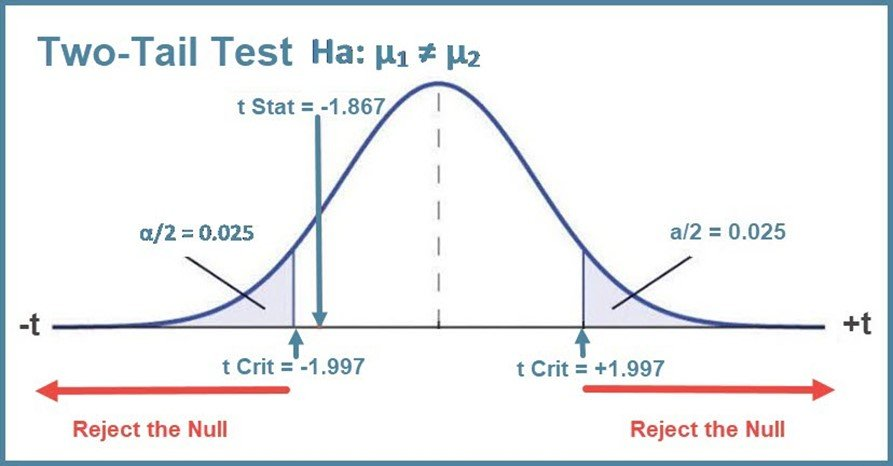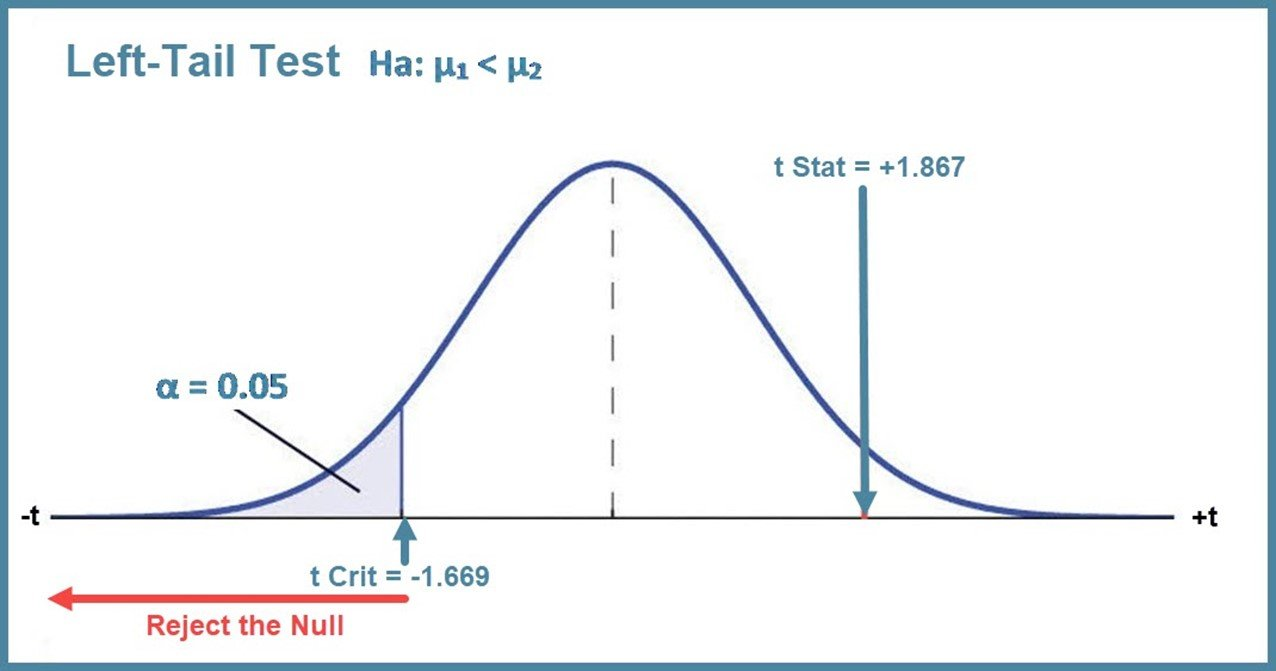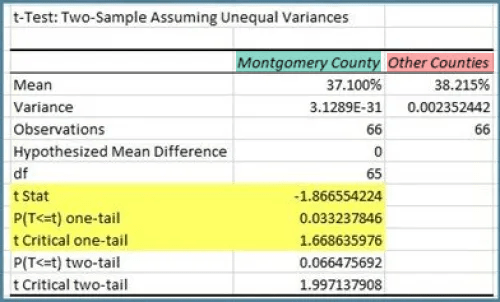# Tail of the Test: Interpreting Excel Data Analysis t-test output

Excel’s Data Analysis ToolPak has three tools for running tests of hypotheses using the t-distribution – t-tests. The output from the tools can be a bit confusing because, unlike other statistical software, these do not allow you to specify the “tail of the test” before you run the analysis. Here is how Microsoft explains how to interpret the output here:

“Under the assumption of equal underlying population means, if t < 0, “P(T <= t) one-tail” gives the probability that a value of the t-Statistic would be observed that is more negative than t. If t >=0, “P(T <= t) one-tail” gives the probability that a value of the t-Statistic would be observed that is more positive than t. “t Critical one-tail” gives the cutoff value, so that the probability of observing a value of the t-Statistic greater than or equal to “t Critical one-tail” is Alpha.
“P(T <= t) two-tail” gives the probability that a value of the t-Statistic would be observed that is larger in absolute value than t. “P Critical two-tail” gives the cutoff value, so that the probability of an observed t-Statistic larger in absolute value than “P Critical two-tail” is Alpha.

Understanding what all that means can be a bit daunting. Here is my attempt as a simpler explanation.

For convenience, we are just using the output from the t-test: Two-Sample Assuming Unequal Variances, but the concepts apply to all three t-test tools. And to both one-sample and two-sample tests.

I will present examples of the 5 situations you can encounter.

#### Example 1: Two-tail Test

Montgomery County is where the capital of Alabama is located. Traditionally, the county bridges between urban and rural Alabama communities. But in more recent times, Montgomery county has been classified as a “metro” county. The Montgomery mayor thinks the obesity prevalence in Montgomery County is significantly different from the average obesity prevalence in the other Alabama counties. Note: The sample data is from the National Health Interview Survey (About the National Health Interview Survey, 2019)

State the Null and Alternative hypotheses:

Null Hypothesis Ho: Obesity Prevalence Montgomery County = Mean Obesity Prevalence Other Counties

Alternative Hypothesis Ha: Obesity Prevalence Montgomery County Mean Obesity Prevalence Other Counties

Note: The tail of the test is indicated by the math operator in the Alternative which is always a form of inequality: <, ≠, > . The Not equal (≠) symbol does not “point” to ether side, so this is a two-tail test.

Important: Put the data ranges for the two groups in the in the same relationship as stated in the Alternative Hypothesis. This will help you keep “things” straight and make interpreting the results easier.

The Montgomery County sample (green highlight) is on the left side of the Null equation and should be placed in the Variable 1 Range field. The Other Counties group (red highlight) is on the right side of the Null equation and must be in the Variable 2 Range field. [your data can be anywhere in your worksheet, but it may be better to arrange it in the right relationship there as well.]Here is the output from the Excel t-Test Two-sample Assuming Unequal Variances tool using a significance level alpha, α, of = 0.05.

Note that Montgomery County is on the left [Variable 1 range] and the Other Counties group is on the right [Variable 2 range] in the output. In each of the following examples, I will place the samples data ranges in the Excel dialog box to match their relationship in the hypotheses.We can see that the Montgomery County prevalence is smaller than that of the average of the Other Counties. But is the apparent difference statistically significantly different?

To make our decision on rejecting (or not rejecting) the null, we can look at the three output values we have highlighted in yellow: the t statistic (t Stat), the two-tail p-value, and the two-tail critical value of t.

Why two-tail? Consider this graphic of the above test results:The first rule for deciding whether to reject the Null tells us to compare our test statistic to the critical value.

When we have a two-tail test, we must put half of our significance level α of 5% in each tail to account for the possibility of our test statistic being either positive or negative, i.e. one sample mean being larger or smaller than the other. Putting 2.5% in each tail, we find two critical values: – 1.997 and + 1.997.
Excel gives us the absolute value of the critical values – it ignores the sign or direction of the value and just uses the magnitude.  That means t Critical is -1.997 on the left side and +1.997 on the right side.

If our test statistic, the t Stat, falls in either rejection area, less than – 1.997 or larger than + 1.997, we must reject the Null. But here our t Stat of – 1.867 does not fall in either rejection area, so we must decide to not reject the Null. [an explanation of how the t-stat is calculated is not included here but the process is similar to finding a z-score.]

Another method to decide whether or not to reject the Null is to compare the two-tail p-value against our significance level. This is labeled “P(T<=t) two-tail.” Thankfully, for a two-tail test, we can always just use the two-tail p-value the Excel tool gives us. It is 0.066 which is larger than our significance level, alpha, of 0.05. Thus, this rule also tells us to not reject the Null that there is no difference in the ratings.

Note that the two rules always agree, unless your technology tool is faulty, which is very rare.

For this two-tail test, we do not reject the Null and we conclude that there is no statistically significant difference in the Obesity Prevalence in Montgomery County and the mean obesity prevalence of the other counties in Alabama.

#### Example 2: Left-tail Test – Negative t Stat

If the Mayor believes Montgomery has a lower mean obesity prevalence, we should run a left-tail test. Why not just run the two-tail? As you will see, a one-tail test gives us more “power” to detect a real effect that is there in the direction we believe it to have. The downside of a one-tail test, if you guess wrong and the effect is in the other direction, the test has no power to detect it.

Null Hypothesis Ho: Obesity Prevalence Montgomery County >= Mean Obesity Prevalence Other Counties

Alternative Hypothesis Ha: Obesity Prevalence Montgomery County < Mean Obesity Prevalence Other Counties

Here is our output again with the one-tail values we need, highlighted in yellow.The tail of the test is always determined by the math operator in the Alternative hypothesis, which in this example is the less than symbol. Remember the less than symbol < points to the left, so this is a left-tail test.

Here is our left-tail graphic:Excel reports the absolute value of the critical values.  For a left-tail test, we need the negative t Critical one-tail which is -1.669. You should note that the one-tail critical value is “smaller” than the two-tail value of -1.997 because we put all of the alpha in that one tail which “pushes” the critical value toward the mean.

Now, the t Stat does fall in the rejection area, so the results say we must reject the Null hypothesis.

To use the second rule, we need to determine the one-tail p-value. Here, Excel’s output can be confusing. If the t Stat is positive, the Excel one-tail p-value is for the right tail – the probability of getting a positive value for t-stat that is as large or even larger.

If the t Stat is negative, the one-tail p-value is for the left tail – the probability of getting a value for t-stat that is as small (negative) or even smaller (more negative).

Here, the t Stat is negative, so the one-tail p-value is for the left tail test, which is what we need.  It is 0.033 which is less than our alpha of 0.05.

So, this result also tells us to reject the Null and we conclude the Obesity Prevalence in Montgomery County is significantly less than the Mean Obesity Prevalence of the other Alabama counties.

Important: You should notice that for the same sample data, the left tail test had to power to reject the Null while the two-tail test did not. You should also notice that the one-tail p-value is exactly half of the two-tail p-value. This is always true.

#### Example 3: Left-tail test – Positive t Stat

Let’s assume the State Public Health Officer claims that the average obesity prevalence in Alabama counties is less than that in Montgomery county.

The null and alternative are:

Null Hypothesis Ho: Mean Obesity Prevalence Other Counties >= Obesity Prevalence Montgomery County

Alternative Hypothesis Ha: Mean Obesity Prevalence Other Counties < Obesity Prevalence Montgomery County

Here is the output of the t-test. Note that the Other Counties Data has been placed In Variable 1 (left) location to match its location in the Alternative Hypothesis.Because the t Stat is positive, the Excel one-tail p-value is for the right tail test, which is the area under the curve above ( to the right of) the t Stat of 1.867. We need to find the complement (the area to the left of t Stat) to use it for the left tail test here. So, the left-tail p-value is 1 – 0.0332 = 0.9676. That is much larger than 0.05, so this method tells us to not reject the Null.

Remember the critical value for the left tail test is -1.669. Our t-stat of +1.867 does not fall in the rejection area to the left of -1.669, so this method also, as you should expect, tells us not to reject the Null.

We conclude the State Health officer’s claim that the average obesity prevalence of the rest of the state is significantly less than Montgomery County’s is not supported by the evidence.

#### Example 4: Right-tail test – Negative t Stat

Now our Mayor claims Montgomery County has a higher obesity prevalence than the average of the other Alabama counties.

Null Hypothesis Ho: Obesity Prevalence Montgomery County <= Mean Obesity Prevalence Other Counties

Alternative Hypothesis Ha: Obesity Prevalence Montgomery County > Mean Obesity Prevalence Other Counties

Remember the tail of the test is indicated by the math operator in the Alternative. Here, the Alternative math operator is greater than > which points to the right, so this is a right-tail test.

Again, we place the data for the two samples to match their relationship in the hypotheses. Here is the highlighted one-tail t-test results:Here is our graphic:For a right-tail test, we are interested in what is happening on the right side of the curve. We use the positive one-tail critical value of +1.669 and we find our t Stat of -1.866 is very far away from the right tail rejection area. So, the first rule tells us to not reject the Null.

Because our t Stat is negative, the one-tail p-value is for the left-tail test. To find the right-side p-value, we must recall that the area under the curve is equal to 1. We want the right-tail p-value, so we must subtract that left-tail value from 1 to get the right-tail p-value. 1 – 0.0344 = 0.966, which is much larger than 0.05, so this rule tells us to not reject the Null of no difference in the ratings of the two groups.

We conclude that there is insufficient evidence to support the claim that Obesity prevalence in Montgomery is significantly greater than the average of the other Alabama counties.

#### Example 5: Right-tail – Positive t-stat

Finally, the State Public Health Officer claims that the average obesity prevalence in Alabama counties is greater than that in Montgomery county.

The null and alternative are:

Null Hypothesis Ho: Mean Obesity Prevalence Other Counties <= Obesity Prevalence Montgomery County

Alternative Hypothesis Ha: Mean Obesity Prevalence Other Counties > Obesity Prevalence Montgomery County

Here is the output of the t-test. Note that the Other Counties Data has been placed In Variable 1 (left) location to match its location in the Alternative Hypothesis.Using the p-value method, we see the t Stat is positive. That means the Excel one-tail p-value is for the right-tail test and we can use it directly to decide to reject the Null, the p-value of 0.033 < 0.05.

And our t-stat of +1.887 is greater than the right tail critical value of +1.669, so that too tells us to reject the Null of no difference. We conclude that there is sufficient evidence to support the claim that the mean obesity prevalence in the other Alabama counties is significantly greater than the prevalence in Montgomery county.

#### Summary

It is important to note, in the 2nd example, that while using the left-tail test gave us the power to detect the significant “less than” difference between the prevalences, using the right-tail test does not detect any difference. That is why you need to be careful if you decide to use a one-tail test and be pretty sure of the direction of the difference. Using a two-tail test is a bit more conservative in that it will pick up a larger difference either way but misses the smaller significant “less than” difference on the left side.

Using the proper tail of the test makes all the difference.

References

Support.Office. (n.d.). Use the Analysis ToolPak to perform complex data analysis. Retrieved from Support.Office: http://bit.ly/2XXgg6T

### 10 thoughts on “Tail of the Test: Interpreting Excel Data Analysis t-test output”

1.Thank you so much for this comprehensive yet concise explanation! It really helped me understand the differences between the tests.

2.thank you so much, I looked almost all day for an explanation like this and am so glad I found it!

3.This is very, very helpful! You’re explanation is so easy to understand especially for people not knowledgeable at stat like me 😀

4.Thanks for the positive feedback.

5.Great job, Dawn!! Seriously… clean and clear! 🙂

6.Very good. Enjoyed the way you explained using worked examples.

7.Was confused between methods to reject null hypothesis and here both methods are present together which cleared the things.

8.I could not remember about how to interpret t-test results according to whether I was performing a left- or right-tailed test. (How do I know which tail the t-statistic is calculated for?) Your explanation was extremely clear and helpful. Thank you!

9.Great explanation, Thank you.

10.Thanks for your detailed explanation. This can be a really complex area and you’ve explained it well. I also use the Pearson correlation for paired data which is another powerful tool.

This site uses Akismet to reduce spam. Learn how your comment data is processed.